# Ace

From complete sets of playing cards (32 cards), we pulled out one card.

What is the probability of pulling the ace?

Result

p =  12.5 %

#### Solution:

$p = \dfrac{ 4}{32} \cdot 100 = 12.5 \%$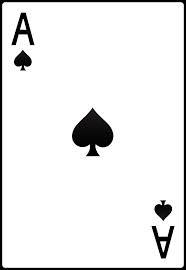Our examples were largely sent or created by pupils and students themselves. Therefore, we would be pleased if you could send us any errors you found, spelling mistakes, or rephasing the example. Thank you!

Leave us a comment of this math problem and its solution (i.e. if it is still somewhat unclear...):Be the first to comment!Tips to related online calculators
Would you like to compute count of combinations?

## Next similar math problems:

1. Christmas holidays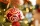How many percent take Christmas holidays lasting 18 days from a school year that lasts 303 days? Round the result to two decimal places
2. Triangular prismThe base of the perpendicular triangular prism is a rectangular triangle with a hypotenuse of 10 cm and one leg of 8 cm. The prism height is 75% of the perimeter of the base. Calculate the volume and surface of the prism.
3. The manufacturer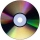The manufacturer found that 3% of the plates produced had a malfunction. Of the compliant, 75% are first and 25% second. What is the probability of producing first and second class plates?
4. The testThe test contains four questions, and there are five different answers to each of them, of which only one is correct, the others are incorrect. What is the probability that a student who does not know the answer to any question will guess the right answer
5. Corona again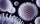If the population on long island is 7.75 million and the coronavirus has affected 22,178. What percent of the population is affected. (looking for 1 in? If possible)
6. Price reductionThe product is sold for 360 CZK and the sales profit is 30%. By what percentage the sales profit will be reduced if I reduce the price of the product by 10%?
7. Rectangular land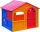On a rectangular land with dimensions of 35 m and 18.5 m is a house with a square floor plan with a side of 14 m. What % of the land is not occupied?
8. What percent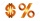What percent of 51 3/5 is 47 2/5?
9. ProbabilityHow probable is a randomly selected three-digit number divisible by five or seven?
10. Cllub flag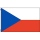The children had the club flag sewn on. The red color covered 2/20 of the total area of the flag, the blue area a fifth, the yellow 19% of the total area. What% is the green area occupied?
11. ChestnutsThree divisions of nature protectors participated in the collection of chestnut trees.1. the division harvested 1250 kg, the 2nd division by a fifth more than the 1st division and the 3rd division by a sixth more than the second division. How many tons of
12. Cone roofHow many m2 of roofing is needed to cover a cone-shaped roof with a diameter of 10 m and a height of 4 m? Add an extra 4% to the overlays.
13. ContestantsThe three best contestants are to divide the total prize of CZK 4,200. The second gets 20% more than the third. And the first one gets 200 CZK less than the second and the third together. How much will everyone get?
14. In the classroomThere are 30 boys and a few girls in the class. In the six months, 28 boys and all girls benefited, which was 95% of all pupils. How many pupils are there in the classroom?
15. KostkaKostka je vepsána do koule o poloměru r = 6 cm. Kolik procent tvoří objem kostky z objemu koule?
16. The surface areaHow much percent will the surface area of a 4x5x8 cm block increase if the length of the shortest edge is increased by 2 cm?
17. Corona virus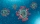Calculate an estimate of the percentage of GDP (Gross Domestic Product) declined due to the quarantine related to the corona COVID-19 virus that lasts for 2 months, during which time the economic activity is down by 80%.